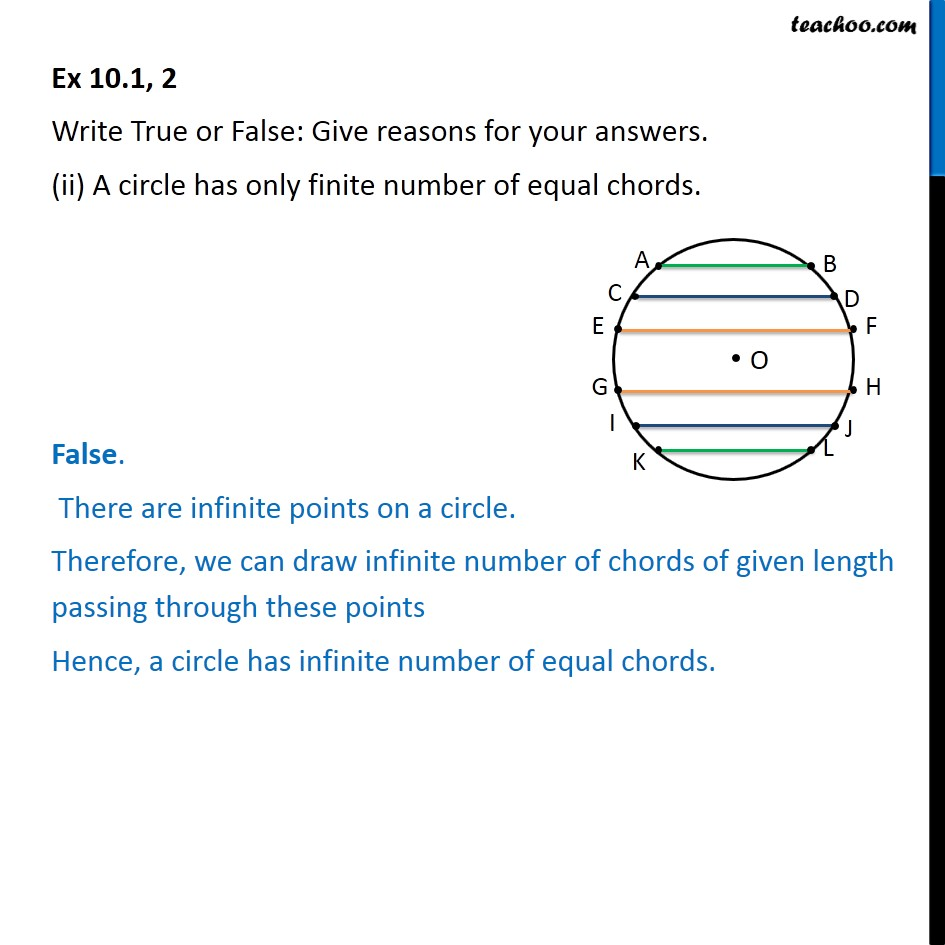Circle - Basic Definitions

Chapter 9 Class 9 Circles
Serial order wiseLearn in your speed, with individual attention - Teachoo Maths 1-on-1 Class

### Transcript

Question 2 Write True or False: Give reasons for your answers. (ii) A circle has only finite number of equal chords. False. There are infinite points on a circle. Therefore, we can draw infinite number of chords of given length passing through these points Hence, a circle has infinite number of equal chords.